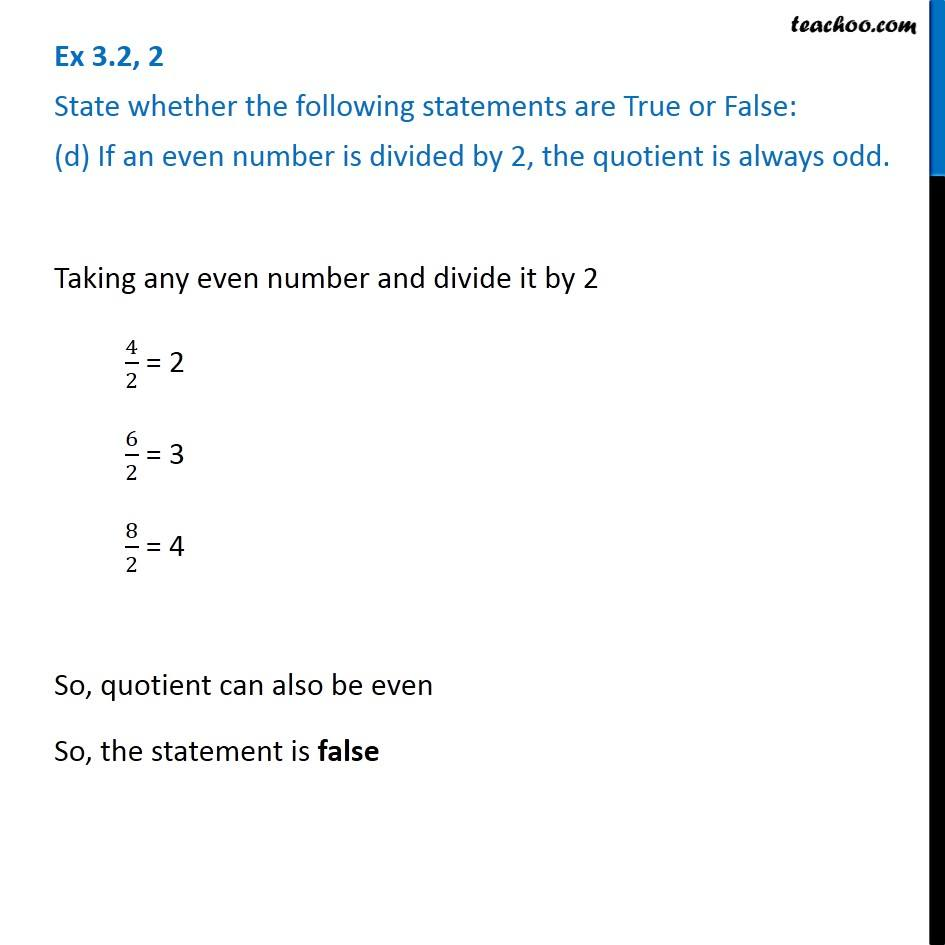Ex 3.2

Chapter 3 Class 6 Playing with Numbers
Serial order wiseLearn in your speed, with individual attention - Teachoo Maths 1-on-1 Class

### Transcript

Ex 3.2, 2 State whether the following statements are True or False: (d) If an even number is divided by 2, the quotient is always odd. Taking any even number and divide it by 2 4/2 = 2 6/2 = 3 8/2 = 4 So, quotient can also be even So, the statement is false Ex 3.2, 2 State whether the following statements are True or False: (e) All prime numbers are odd. The Prime number 2 is even So, statement is False